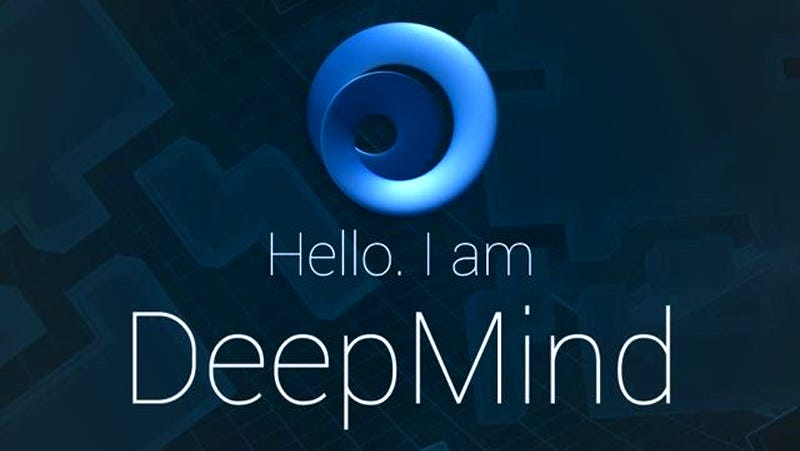# Ai breakthrough -NALU

Even if deep networks have evolved so far, neural networks were unable to count numbers till now, but DeepMind’s recent paper published by Trask, made this possible.

He introduced a unit called NALU(neural arithmetic logical units), which can extrapolate the mechanism/relation between the numbers outside they have been trained with. It turns out to be an attention mechanism as shown below.

For the first time in history of deep learning, a nn learns concept of a number deciding which part of input & output should have certain functions applied.

There is a NAC-neural accumulator whose weight matrix consists of only -1s,0s,1s and outputs are addition/subtraction of input vectors instead of rescaling which prevents layers from rescaling when mapping input to the output.

The NAC will multiply weights-W with our input to get output-a, where W is tanh activation of W(hat) multiplied by sigmoid of M(hat) (#we can use SGD here)

A more robust extension of NAC is NALU which includes all complex arithmetics where each complex calculation is performed by each subcell.

So, just like other GRUs & LSTMs, NALU is a network module which has made a breakthrough in learning neural networks.

The paper shows they have experimented NALU on a couple of different tasks: 1. Select inputs and use different functions on them (like +,-,*,/,x²) and it extrapolated well to a new data.

2. Trained it to show how many types of images in mnist data,& again it performed well ( u can see detailed outcome in the paper)

3. Translate a text number expression to a scalar value(eg.FOURTY-FIVE=45) (LSTM+NALU achieved best extrapolation)

Thus, NALU have the ability to truly understand what numbers mean and the interaction between them which is extraordinary and so has a great future with extending its domains.

Credits to Siraj_Raval : http://www.sirajraval.com/…..I’ve merely created a wrapper to understand.